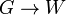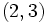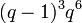# Bruhat decomposition for general linear group of degree three over a field

This article describes the details of the Bruhat decomposition for the general linear group of degree three over a field$K$. Let$G =GL(3,K)$ and$B$ denote the Borel subgroup of upper-triangular matrices. We have that:$G = \bigcup_{w \in W} BwB$

where$W$ is the Weyl group, which in this case can be identified with symmetric group:S3. In other words, there is a set map$G \to W$ whose fibers are the double cosets of$B$, and whose restriction to the subgroup$W$ of$G$ is the identity map. The map is well defined because every double coset of$B$ intersects$W$ at a unique point.

Note that the set map is not a homomorphism of groups.

Another way of putting this is that there is a set map from the left coset space$G/B$ to$W$ that sends a left coset containing an element of$W$ to that element of$W$, and that is invariant under the left action of$B$ by multiplication.

## Interpretation in terms of flags

The mapping:$G/B \to W$

can be interpreted as follows: an element of$G/B$ is a complete flag of subspaces for the three-dimensional space$K^3$, and the mapping to$W$ describes its relative position with respect to the standard flag (the one stabilized by$B$). If the flag is equal to the standard flag, then the map sends it to the identity element of$W$, otherwise it is sent to one of the non-identity element of$W$. The generic flag gets sent to the anti-diagonal permutation, corresponding to$(1,3)$.

## Finite field case

In the finite field case, for a finite field with$q$ elements, the fibers for the Bruhat map:$G/B \to W$

can be computed explicitly.

Element of$W$ Expression in Bruhat terms Matrix Size of fiber in$G/B$ (equals$q$ to the power of the Bruhat word length) Degree of polynomial (= word length of Bruhat word) Size of fiber in$G$ Explanation$()$ empty word$\begin{pmatrix} 1 & 0 & 0 \\ 0 & 1 & 0 \\ 0 & 0 & 1 \\\end{pmatrix}$ 1 0$(q-1)^3q^3$$(1,2)$$s_1$$\begin{pmatrix} 0 & 1 & 0 \\ 1 & 0 & 0 \\ 0 & 0 & 1 \\\end{pmatrix}$$q$ 1$(q-1)^3q^4$$(2,3)$$s_2$$\begin{pmatrix} 1 & 0 & 0 \\ 0 & 0 & 1 \\ 0 & 1 & 0 \\\end{pmatrix}$$q$ 1$(q-1)^3q^4$$(1,2,3)$$s_1s_2$$\begin{pmatrix} 0 & 0 & 1 \\ 1 & 0 & 0 \\ 0 & 1 & 0 \\\end{pmatrix}$$q^2$ 2$(q - 1)^3q^5$$(1,3,2)$$s_2s_1$$\begin{pmatrix} 0 & 1 & 0 \\ 0 & 0 & 1 \\ 1 & 0 & 0 \\\end{pmatrix}$$q^2$ 2$(q - 1)^3q^5$$(1,3)$$s_1s_2s_1$$\begin{pmatrix} 0 & 0 & 1 \\ 0 & 1 & 0 \\ 1 & 0 & 0 \\\end{pmatrix}$$q^3$ 3$(q - 1)^3q^6$
Total (6 rows) -- --$q^3 + 2q^2 + 2q + 1 = (q + 1)(q^2 + q + 1)$ equals size of$G/B$ --$q^3(q-1)^3(q + 1)(q^2 + q + 1)$ equals order of$G$